# Hydrogenaudio Knowledgebase:Sandbox

some text that is not formated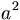$a^2$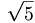$\sqrt{5}$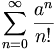$\sum_{n=0}^\infty \frac{a^n}{n!}$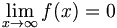$\lim_{x\to\infty}f(x)=0$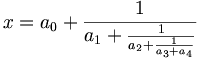$x = a_0 + \frac{1}{a_1 + \frac{1}{a_2 + \frac{1}{a_3 + a_4}}}$

Does things work after update someone wonders...